Skip to main content

Thank you for visiting nature.com. You are using a browser version with limited support for CSS. To obtain the best experience, we recommend you use a more up to date browser (or turn off compatibility mode in Internet Explorer). In the meantime, to ensure continued support, we are displaying the site without styles and JavaScript.

# Information entropy of coding metasurface

## Abstract

Because of their exceptional capability to tailor the effective medium parameters, metamaterials have been widely used to control electromagnetic waves, which has led to the observation of many interesting phenomena, for example, negative refraction, invisibility cloaking, and anomalous reflections and transmissions. However, the studies of metamaterials or metasurfaces are mainly limited to their physical features; currently, there is a lack of viewpoints on metamaterials and metasurfaces from the information perspective. Here we propose to measure the information of a coding metasurface using Shannon entropy. We establish an analytical connection between the coding pattern of an arbitrary coding metasurface and its far-field pattern. We introduce geometrical entropy to describe the information of the coding pattern (or coding sequence) and physical entropy to describe the information of the far-field pattern of the metasurface. The coding metasurface is demonstrated to enhance the information in transmitting messages, and the amount of enhanced information can be manipulated by designing the coding pattern with different information entropies. The proposed concepts and entropy control method will be helpful in new information systems (for example, communication, radar and imaging) that are based on the coding metasurfaces.

## Introduction

The subwavelength nature of metamaterials and/or metasurfaces enables them to be described by effective medium parameters (for example, electric permittivity, magnetic permeability, index of refraction and impedance)1, 2. Because of the flexible designs of meta-atoms and their arbitrary arrangements, the effective medium parameters can be tailored to have extreme values3, 4, high inhomogeneity5, 6 and strong anisotropies7, 8. Governed by the transformation optics9, 10 and other physical principles11, this unique flexibility in design makes metamaterials and metasurfaces very powerful in controlling the electromagnetic waves. Hence, many exciting and unusual phenomena that do not occur in conventional media have been realized in metamaterials and/or metasurfaces, such as negative refraction3, perfect imaging12, invisibility cloaking9, 10, 13, 14, 15, 16, 17, optical and radar illusions18, 19, electromagnetic concentrators20 and rotators21, microwave and optical black holes11, 22, 23, anomalous reflections and transmissions24, 25, optical vortex24, 26, broadband light bending27, photonic spin Hall effect28, polarization traffic controls29, 30, and polarization rotations31. In addition to the observation of exciting physical phenomena, metamaterials have found wide applications in engineering due to their excellent performance, including novel antennas32, 33, microwave components34 and satellite communications.

However, the current studies of metamaterials and metasurfaces have been mainly limited to their physical features and the related phenomena and functional devices, with a lack of viewpoints from the information perspective. Recently, the concepts of coding, digital and programmable metamaterials/metasurfaces have been introduced and experimentally demonstrated35, 36, 37; such concepts can bridge the gap between the metamaterial (or metasurface) and information science. Instead of using the effective medium parameters to describe metamaterials, ‘0’ and ‘1’ coding particles with opposite phase responses have been used to characterize metamaterials. Hence, the digital coding information (or messages) can be directly encoded to the coding metamaterials, which can further be digitally controlled and even programmable35. The manipulations of the electromagnetic waves using the coding metasurfaces have been investigated at both microwave and terahertz frequencies35, 36, 37.

Information theory originally evolved from physics but has since developed rapidly as a new and independent science. Information science is currently a broad interdisciplinary field. According to the definition by Borko38, ‘information science is that discipline that investigates the properties and behavior of information, the forces governing the flow of information, and the means of processing information for optimum accessibility and usability.’ Over the past several decades, information science and technologies have experienced huge improvements and have pushed the developments of modern industries and societies. Hence, it is important to connect information science with metamaterials or metasurfaces, which is one of the most attractive topics in physics. In this article, the goal is to answer the following questions: (1) How can one measure the information of a metasurface? (2) How does one measure the information of the physical features of metasurface? (3) Can a metamaterial or metasurface enhance information capacities? (4) Finally, how does one manipulate information using a metasurface? Here we focus on studying coding metasurfaces because they can directly interact with the coding information. However, the proposed concepts, methods and interpretations can be easily extended to general metasurfaces and metamaterials.

## Materials and methods

In information theory, any system is composed of transmitters, receivers and channels. The transmitter is used to generate messages, which are modulated by the channel and finally sent to the receiver. In this context, Shannon entropy is the average value of the information carried in each message39. Without loss of generality, we consider a reflection-type information system, as shown in Figure 1a, in which the electromagnetic waves containing the messages sent by the transmitter are reflected by a perfectly electrical conductor (PEC). Through the free-space channel, the modulated electromagnetic waves are captured by the receiver in the far-field region. Here the PEC reflector is part of the channel for message transmission. If the PEC reflector has infinite size, then total reflection will occur; if it has a finite size, there will be a scattering effect, as discussed below.Figure 1

In fact, we can use coding metasurfaces to replace the PEC reflector to further modulate the channel and enhance the information carrier, as demonstrated in Figure 1b. Through the coding metasurfaces, we could control the information sent by the same transmitter. To investigate the information modulation quantitatively, we consider a general case of a 1-bit coding metasurface, whose electric current density is expressed as J(x,y)=J0ei ϕ0, for coding digit ‘0’; J(x,y)=J0ei(ϕ0+π), for coding digit ‘1’. Here J0 and ϕ0 are constants. After a simple derivation (Supplementary Information), we easily show that the far electric fields can be written in a closed form as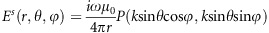in which r, θ and ϕ indicate the distance, elevation angle and azimuth angle in spherical coordinates, respectively, k is the wavenumber in free space and P(ksinθcosϕ, ksinθsinϕ) is the Fourier transformation of the electric current distribution on the coding metasurface. Note that the far electric field of the coding metasurface (that is, the physical information) is just the Fourier transform of the coding pattern (that is, the geometrical information).

Next, we study the geometrical information and physical information of coding metasurfaces quantitatively. To describe the geometrical (or coding pattern) information, we adopt the normalized Shannon entropy defined as H1=−N−1xP(x)log2P(x), in which x{0,1}N, N is the number of coding units and P(x) represents the corresponding probability. Clearly, the entropy H1 reaches its maximum value of 1, as the appearances of digital states 0 and 1 on the coding units have equal possibility of 1/2 independently. Usually, the metasurface units are programmed in a clustered manner, and the neighboring metasurface units are dependent on each other. For simplicity, we adopt the anisotropic Markov random field to model the probability function P(x). As a consequence, the entropy reads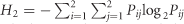for a pair of units indexed by i and j, in which Pij is the joint probability of a group G(i, j) representing two adjacent coding elements, as shown in Figure 1c. For any coding metasurfaces, there are four different cases: G(0, 0), G(0, 1), G(1, 0) and G(1, 1). The probability of the appearance of these four cases determines the two-dimensional (2D) information entropy of a coding metasurface. From Figure 1c, we note that the adjacent code in group G(i, j) can be on either the right side (GR(i, j)) or the upper side (GU(i, j)) of the current code (indicated by the red color). Hence, we should first calculate the 2D entropies of the coding pattern when the adjacent pixel is considered in the row (H2R) and column (H2U), after which we can arrive at the information entropy approximated by H2ave=(H2R+H2U)/2 up to a constant.

Figure 1d presents the processes to calculate the physical entropy of a coding metasurface from its far-field pattern. As discussed above, the far-field pattern can be directly obtained by the fast Fourier transform (FFT) of the coding pattern. However, a coordinate transformation is required to obtain the image of final far-field pattern in the 2D polar coordinate system from the original FFT image (see Supplementary Information for details). On the basis of the image of far-field pattern, the physical entropy of a coding metasurface is expressed as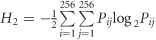, in which Pij represents the joint probability of a group G (i, j): the gray level i of the current pixel and the gray level j of its adjacent pixel. The physical entropy of a coding metasurface can directly estimate the average amount of information of each pixel in its far-field pattern image.

## Results and discussion

Using the above-defined geometrical and physical entropies, we reconsider the reflection-type information system. The PEC reflector is in fact a full-‘1’ coding metasurface35, as shown in Figure 2a(i), which has zero geometrical entropy due to the constant coding sequence. The FFT, polar far-field images and three-dimensional (3D) far-field pattern of the PEC reflector are illustrated in Figure 2a(ii)–2a(iv), from which the physical entropy is calculated as 0.9273. Here the electric size of the PEC reflector is 14.93 × 14.93 λ2 (λ is the free-space wavelength). This physical entropy can be considered the basic far-field information of the reflection system with a certain size. We remark that the physical entropy of the PEC reflector will decrease as the size increases. Ideally, in the case of infinite PEC reflector, the far-field pattern will be a Dirac delta function, and the physical entropy will thus approach zero.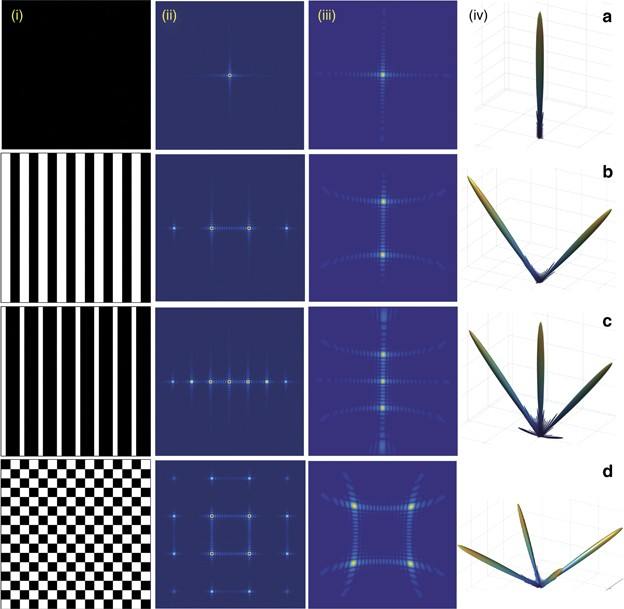Figure 2

For the same-sized coding metasurface, we use different coding sequences to control both the geometrical and physical entropies. Figure 2b–2d demonstrates three cases of periodic coding patterns and their far-field features. In both Figure 2b(i) and 2d(i), ‘0’ and ‘1’ coding particles appear equally in quantity; hence, the geometrical entropy will be 1 if the original Shannon’s definition (H1) is adopted. However, such two coding distributions are apparently different, and we should thus use H2 entropy to measure their geometrical information. For the case in Figure 2b(i), if the probabilistic model considers individual codes to be independent, then H2=0.7028. Nevertheless, if the coding sequence is considered a 2 × 2 matrix (0,1; 0,1) periodically with four codes as a symbol, then the entropy becomes zero. Therefore, there are only four independent codes in this periodic case, and the total geometrical information is 4 × 0.7028=2.8112. Because the total number of periodic blocks on the coding metasurface is 8 × 8=64, the average geometrical entropy should be 2.8112/64=0.0439. The corresponding FFT image and far-field patterns are demonstrated in Figure 2b(ii)–2b(iv), and the physical entropy is calculated as 1.3923. Compared with the case of PEC reflector shown in Figure 2a, we notice that the physical entropy of the coding metasurface is increased. This is apparent because the metasurface in this case radiates two beams (Figure 2b(iv)), which naturally have more information than the single beam radiated by the PEC reflector (Figure 2a(iv)). For the other two periodic coding cases presented in Figure 2c and 2d, the average geometrical entropy and physical entropy are given in Table 1. We clearly observe that the coding sequence 011101110111… (with the average geometrical entropy of 0.0369) produces three far-field beams (Figure 2c(iv)), which contain more information (with the physical entropy of 1.8047) than the two-beam pattern; the chess-board coding (with the average geometrical entropy of 0.0566) generates four far-field beams (Figure 2d(iv)), which possess more information (with the physical entropy of 2.0467) than the three-beam pattern.

We further study the information entropies of non-periodic coding metasurfaces. Figure 3a–3c(i) shows three typical non-periodic coding patterns, that is, Jerusalem-cross, circular-ring and random codes, in which the probabilistic model of individual code is independent, resulting in larger geometrical entropy. Here the white and black areas indicate ‘0’ and ‘1’ coding particles, respectively. Interestingly, Figure 3a and 3b shows that the 2D polar far-field pattern (Figure 3a(ii)) of the Jerusalem-cross coding also looks like a Jerusalem cross; in contrast, the 2D polar far-field pattern (Figure 3b(ii)) of the circular-ring coding is composed of a series of circular rings and a Jerusalem cross. The 3D far-field patterns shown in Figure 3a–3b(iii) present clearly designed functionalities: the Jerusalem-cross coding produces a main beam and four side beams directing to different angles, and the circular-ring coding produces four main beams and surrounding sides beams.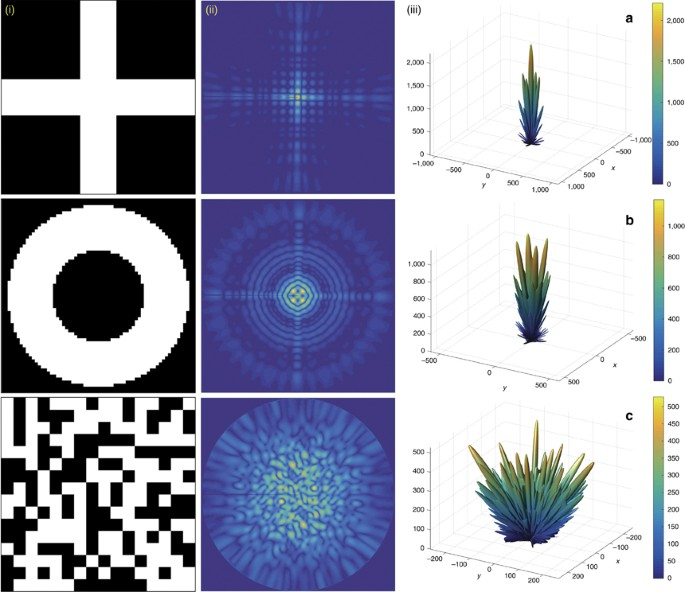Figure 3

In a specific non-periodic coding metasurface, the areas of ‘0’ or ‘1’ particles will also have significant impact on the information entropy. Supplementary Fig. S2 illustrates three Jerusalem-cross codes, in which the ‘0’ coding particles gradually increase from Supplementary Fig. S2a to S2c; the corresponding geometrical entropies are 0.2474, 0.3522 and 0.4798, respectively. The results indicate an increase in the amount of geometrical information. In all three cases, the 2D polar far-field patterns look like three Jerusalem crosses of different dimensions (Supplementary Fig. S2a–2c(ii)), and the 3D far-field patterns always have a main beam surrounded by multiple side beams with different levels (Supplementary Fig. S2a–2c(iii)), in which the corresponding physical entropies are 1.5996, 1.7200 and 2.1795. The study of circular-ring codes with increasing ‘0’-particle areas is presented in Supplementary Fig. S3, in which the geometrical entropies for cases a, b and c are 0.5744, 0.5111 and 0.6193, respectively, and the physical entropies are 2.4144, 2.3485 and 3.2114, respectively. It is interesting to find that the information entropy in this specific case does not increase monotonically as the ‘0’ area increases. However, when the geometrical entropy decreases, the physical entropy also decreases, as clearly illustrated in Table 1.

An extreme example for non-periodic coding is the random pattern, as depicted in Figure 3c(i), in which the geometrical entropy reaches the very high level of 0.7780. For the random coding pattern, the 2D polar far-field pattern is also randomly distributed (Figure 3c(ii)), which results in a large physical entropy of 4.6413 and implies significantly enhanced information. The 3D far-field pattern in Figure 3c(iii) clearly demonstrates the diverse beam radiations or scattering, which carry much more information than the above-described regular (or simple) patterns with fewer beams. For comparison, the geometrical and physical entropies for the random coding pattern are listed in Table 1. Considering all examples shown in Table 1, we observe that the physical entropy and geometrical entropy have an approximately monotonic relation. In most cases, when the geometrical entropy for coding pattern increases, the physical entropy for far-field pattern becomes higher.

To quantitatively explore the relationship between the geometrical and physical information entropies, we consider a large number of random coding patterns, which are generated by the model of cellular automata machine40, mimicking the diffusion process of gas molecules (Supplementary Information). At the initial state shown in Figure 4a(i), the coding metasurface is composed of 64 × 32 coding particles of ‘1’ (on the left side) and 64 × 32 coding particles of ‘0’ (on the right side) that are totally separated from each other, forming a 64 × 64 coding metasurface with the minimum geometrical entropy. The diffusion of coding patterns is realized by each time randomly selecting two adjacent coding particles and then interchanging them. We set 500 steps of such interchanging operations as one iteration cycle. Figure 4b(i) and 4c(i) gives the random coding metasurfaces generated at the 50th and 99th iterations. Together with the initial state, the far-field patterns are demonstrated in Figure 4a–4c(ii–iii). As the number of iterations increases, from Figure 4a–4c, we note that the coding pattern becomes more random, the 2D polar far-field pattern becomes more diffuse and the 3D far-field pattern has increasing amounts of radiation or scattering beams. The detailed generation process of the random coding metasurfaces is presented in Supplementary Fig. S5. When the iteration increases from 1 to 99 (or the step of interchanging operation increases from 0 to 49 500), the geometrical and physical entropies of the coding metasurfaces are depicted in Figure 4d. As expected, as the number of iterations increases, the geometrical entropy roughly becomes larger (similar to the gas molecules, see Supplementary Information), and the physical entropy generally becomes larger.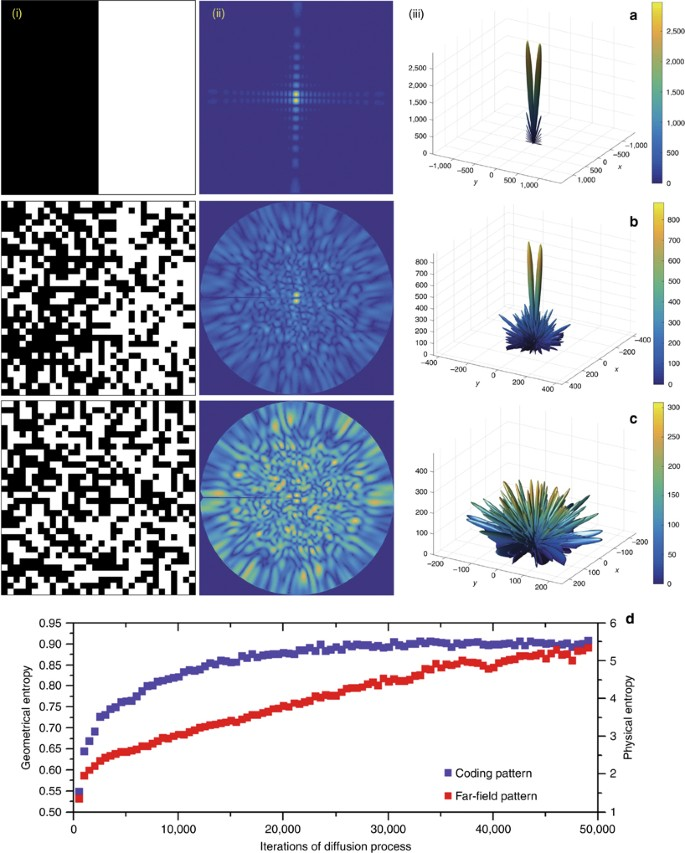Figure 4

It is very important to realize the proposed coding metasurfaces and verify their far-field patterns by full-wave numerical simulations. Hence, we design a metamaterial element, as shown in Figure 5, to realize the ‘0’ and ‘1’ particles. Figure 5a shows the structure of the coding particle, which is composed by printing a metallic square sheet with length L on the top of a substrate with period p=7 mm and thickness d=1.6 mm. The thickness of metallic layer is set as 0.018 mm. The permittivity and loss tangent of the dielectric substrate (FR4) are ɛr=4.3 and δ=0.03. Using the frequency-domain solver in commercial software, the CST Microwave studio, we obtain the amplitudes and phases of eight coding particles at 10 GHz in Figure 5b. These coding particles are labeled as 3-bit coding digits 000, 001, 010, 011, 100, 101, 110 and 111, when the length L equals 7, 6.16, 5.67, 5.38, 5.15, 4.88, 4.43 and 1.26 mm, respectively. Here the adjacent coding particles have a phase difference of π/4, forming a 3-bit reflection-type coding metasurface. Because the backside of the substrate is fully covered by a PEC, the amplitudes of all eight coding particles are beyond 0.84, thus providing a good approximation to ideal coding particles with unity amplitude of reflection. We note that such a 3-bit coding metasurface can be utilized as a 2-bit or 1-bit coding metasurface by simply selecting the coding particles with corresponding phase differences of π/2 and π, respectively.Figure 5

To validate the performance of the proposed metasurfaces and the accuracy of far-field pattern calculated theoretically by FFT, we consider three metasurfaces encoded with the periodic coding sequences 010101…, 01110111… and (0,1;1,0) chess-board, as shown in Figure 2b–2d, respectively. In real structures of coding metasurfaces, the electromagnetic coupling between adjacent unit cells with different geometries will result in different reflection responses from the ideal reflections obtained for a single unit cell placed in the infinitely periodical boundary condition. To minimize this effect, we combine M × M identical coding particles to form a super unit cell, as can be observed from the coding patterns shown in Figures 6,7 and 8b(i). For the first and third cases (Figures 6 and 8), each coding digit includes 4 × 4 identical coding particles; whereas for the second case (Figure 7), each coding digit includes 2 × 2 identical coding particles. Therefore, all three metasurfaces have the same size as 64 × 64, equivalent to 448 × 448 mm2. Figures 6, 7 and 8b(ii) illustrate the numerically simulated far-field patterns in the 2D polar coordinate system for such three cases, all of which are in excellent agreement with the theoretically calculated results given in Figures 6, 7 and 8a(ii). Similarly, the 3D far-field patterns of the theoretically calculated results (Figures 6, 7, 8a(iii)) and the numerically simulated results (Figures 6, 7, 8b(iii)) are in excellent agreement. The full-wave simulations of the realistic coding metasurface structures validate the accuracy of the far-field patterns that are calculated theoretically by FFT.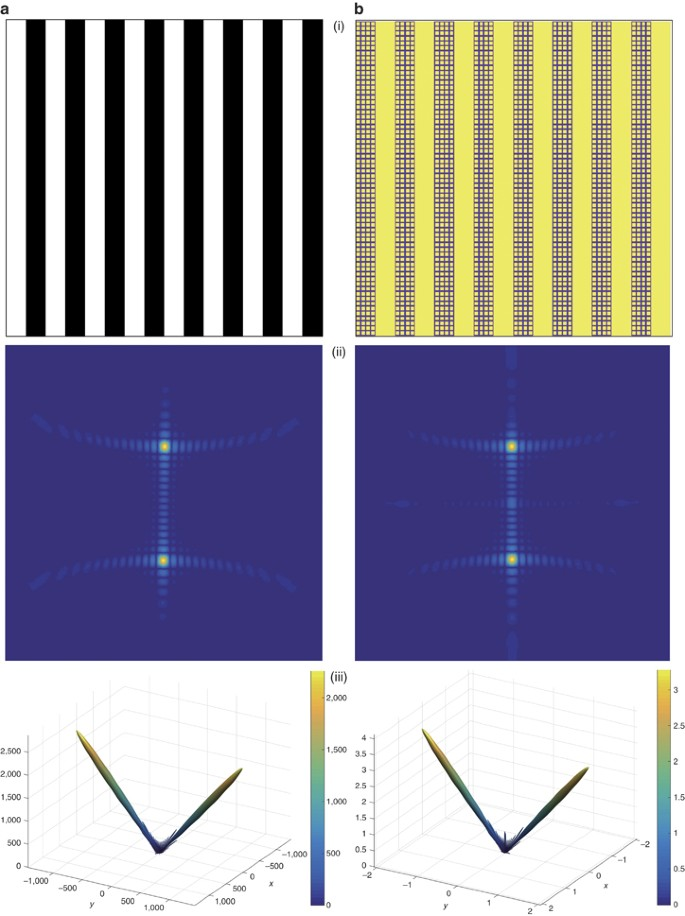Figure 6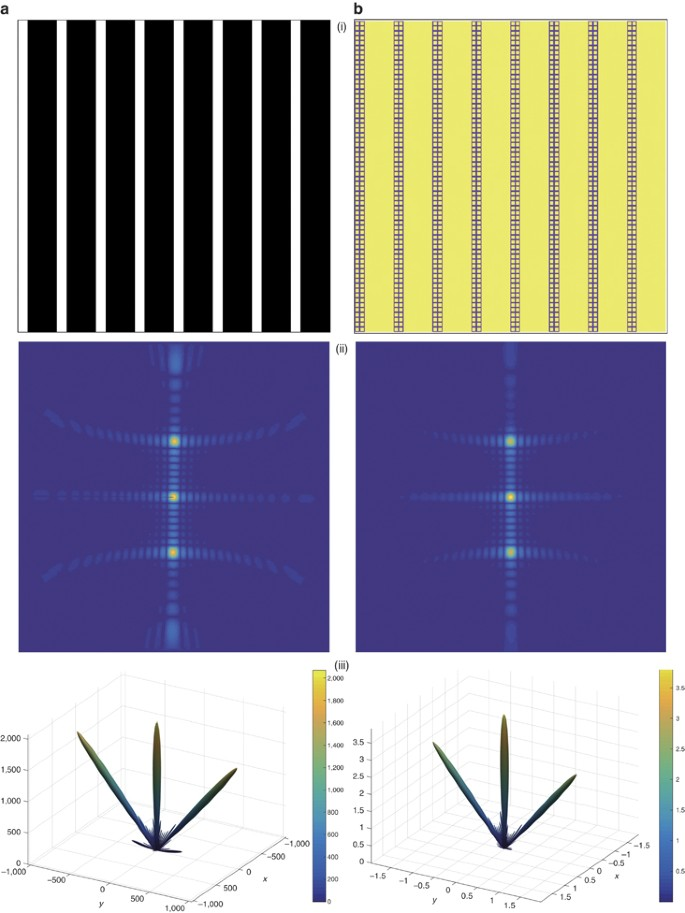Figure 7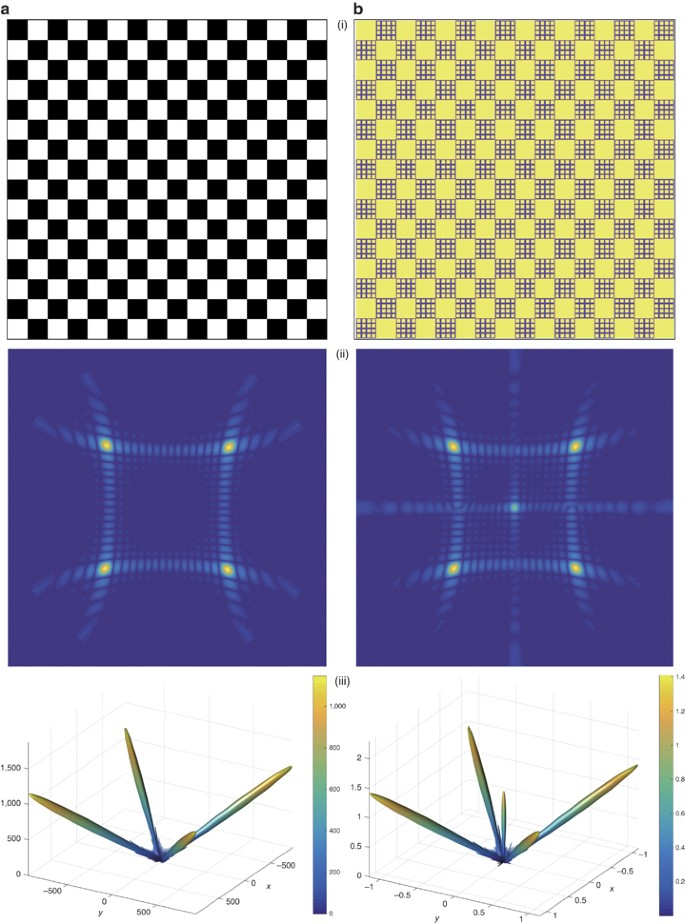Figure 8

As experimental verification of the concept proposed in this work, a sample encoded with periodic sequence ‘010101…’ was fabricated using the standard printed circuit board process on the FR4 substrate (ɛr=4.3+0.129i), as shown in Figure 9a; the sample is composed of 32 × 32 coding particles and covers an area of 224 × 224 mm2. All other parameters of the fabricated sample are kept the same as those in numerical simulations in Figure 6. The photograph of the experimental setup for the measurement of far-field scattering pattern in the horizontal plane is shown in Figure 9b. A horn antenna with working bandwidth from 9.48 to 15 GHz was employed as the feeding antenna to generate the quasi-plane wave for the coding metasurface. Both the feeding antenna and sample were coaxially mounted on a board at a distance of 1.8 m and could automatically rotate 360° in the horizontal plane with high precision. We chose the distance based on the consideration that the feeding antenna should be placed inside the Fraunhofer region of the coding metasurface, which can be calculated by R=2L2/λ, where L is the maximum electric length of the antenna and λ the working wavelength. Substituting L=317 mm and λ=30 mm into the formula, we obtain the required distance R as 3.34 m. This distance ensures that the optical path difference between the center and the edge of the coding metasurface is less than λ/16, providing a quasi-plane wave illumination for the coding metasurface, as is considered in the far-field simulations in CST. As R grows with L2, the distance between the feeding antenna and coding metasurface will become too large for our microwave chamber; thus, the size of the fabricated sample is reduced to half of the model in the simulations. One may notice that the distance 1.8 m in experiment is smaller than the minimum distance R calculated from the formula. We remark that the aforementioned distance of the far-field region was obtained from the assumption of point source excitation, whereas in our experiment the wavefront generated from the horn antenna is relatively flat. Thus, the corresponding distance R could be reduced accordingly.Figure 9

In the experiment, the receiving antenna (not shown in the photograph) automatically recorded the electric fields in the horizontal plane (E-plane) every 0.1° as the board carrying both the feeding antenna and sample rotated from 0° to 360°. Although it is designed to operate at 10 GHz, we obtained a better performance at a slightly larger frequency of 10.5 GHz, as shown in Figure 9c. The frequency shift could be caused by the inaccurate permittivity of the substrate of the fabricated sample. For comparison, we have also provided the corresponding simulation result in Figure 9d at 10 GHz. Note that both the measured and simulated radiation patterns have been normalized to each of their maximum values. We observe that the centers of the scattering peaks of the measured results appear at ±34.1°, in very good agreement with the simulations (±32.1°). In addition, the scattering patterns of the measured and simulated results are highly consistent with each other under the dB scale. Limited by the current experiment condition, we could not measure the 3D scattering patterns for the calculation of entropy. However, given the highly consistency between simulated and measured results, we believe that all the theoretical calculations and predictions are reliable.

The coding metasurface resembles the phase-array antenna or the conventional reflectarray antenna that are widely used in the microwave41, 42 and terahertz spectra43, in the sense that both of them are composed of an array of discrete elements with controllable excitation amplitude and phase, and are capable of producing desired radiation/scattering beams in a flexible manner. However, the metasurface and the phase-array antenna are different in several important aspects. First, the coding metasurface functions as not only an active radiation antenna but also a controllable communication channel. We provide the perspective of information entropy on the coding metasurface and establish theoretically and experimentally the link of information capacity between the coding pattern and its resultant physical response. Our finding indicates that coding patterns with larger entropy could produce more diffuse scattering patterns, that is, possessing more information. This conclusion will serve as a central role in many cutting-edge imaging systems, such as a compressive imaging system, in which the coding metasurface is used as a programmable and expressible lens. Second, the coding metasurface is usually designed with smaller unit cells, that is, typically, λ/8 to λ/4, as opposed to the conventional reflectarrays with the requirement on the size of unit cell not less than half free-space wavelengths. Such a unique property provides the coding metasurface with several advantages over the conventional reflectarray. On one hand, suppose that the size of unit cell of coding metasurface is λ/6; in this case, the whole 6 × 6 identical coding particles could be arranged in a square area of λ × λ, whereas reflectarrays can only accommodate 2 × 2 unit cells in the same area. Thus, the coding metasurface is more powerful than the conventional reflectarray in the sense that we could obtain an accurate expression of the electromagnetic response, which is specially desired in many computational imaging systems. On the other hand, the coding metasurface can be used to perform controllable conversion from the propagating wave to the surface wave (SW) by applying the ‘00 01 10 11…’ coding sequence25 because the size of coding particle is smaller than λ/4. However, the reflectarray is unable to provide sufficient momentum compensation for the normal incidence to be converted to SW because the length of the gradient phase across 2π exceeds λ.

## Conclusions

We showed that the far-field pattern of a coding metasurface is just the Fourier transform of the coding pattern, which provides an analytical connection between the geometrical (coding) and physical (far field) worlds of metasurfaces. This connection was validated by using realistic structures through full-wave simulations. We proposed the use of geometrical entropy to measure the information of the coding pattern and the use of physical entropy to measure the information of far-field pattern. Three types of coding metasurfaces (periodic coding, non-periodic coding and random coding) were presented, demonstrating that the coding metasurfaces can enhance the transmission of information, and the information can be controlled by the coding patterns. We also showed that, as the geometrical entropy increases, the physical entropy generally increases. The proposed concepts and entropy manipulations can find applications in new functional devices and information systems. For example, controlling multi-beam radiations is beneficial to multi-channel communications and multi-target radars, and large amounts of random-beam radiations or scattering are essential to the single-source imaging system44.

## References

1. 1

Smith DR, Pendry JB . Homogenization of metamaterials by field averaging (invited paper). J Opt Soc Am B 2006; 23: 391–403.

2. 2

Liu RP, Cui TJ, Huang D, Zhao B, Smith DR . Description and explanation of electromagnetic behaviors in artificial metamaterials based on effective medium theory. Phys Rev E 2007; 76: 026606.

3. 3

Veselago VG . The electrodynamics of substances with simultaneously negative values of ɛ and μ. Sov Phys Usp 1968; 10: 509–514.

4. 4

Enoch S, Tayeb G, Sabouroux P, Guerin N, Vincent P . A metamaterial for directive emission. Phys Rev Lett 2002; 89: 213902.

5. 5

Kundtz N, Smith DR . Extreme-angle broadband metamaterial lens. Nat Mater 2010; 9: 129–132.

6. 6

Jiang WX, Qiu CW, Han TC, Cheng Q, Ma HF et al. Broadband all-dielectric magnifying lens for far-field high-resolution imaging. Adv Mater 2013; 25: 6963–6968.

7. 7

Chen HS, Wu BI, Zhang BL, Kong JA . Erratum: electromagnetic wave interactions with a metamaterial cloak. Phys Rev Lett 2007; 99: 149901.

8. 8

Lai Y, Chen HY, Zhang ZQ, Chan CT . Complementary media invisibility cloak that cloaks objects at a distance outside the cloaking shell. Phys Rev Lett 2009; 102: 093901.

9. 9

Pendry JB, Schurig D, Smith DR . Controlling electromagnetic fields. Science 2006; 312: 1780–1782.

10. 10

Leonhardt U . Optical conformal mapping. Science 2006; 312: 1777–1780.

11. 11

Narimanov EE, Kildishev AV . Optical black hole: broadband omnidirectional light absorber. Appl Phys Lett 2009; 95: 041106.

12. 12

Pendry JB . Negative refraction makes a perfect lens. Phys Rev Lett 2000; 85: 3966–3969.

13. 13

Schurig D, Mock JJ, Justice BJ, Cummer SA, Pendry JB et al. Metamaterial electromagnetic cloak at microwave frequencies. Science 2006; 314: 977–980.

14. 14

Li JS, Pendry JB . Hiding under the carpet: a new strategy for cloaking. Phys Rev Lett 2008; 101: 203901.

15. 15

Liu R, Ji C, Mock JJ, Chin JY, Cui TJ et al. Broadband ground-plane cloak. Science 2009; 323: 366–369.

16. 16

Ergin T, Stenger N, Brenner P, Pendry JB, Wegener M . Three-dimensional invisibility cloak at optical wavelengths. Science 2010; 328: 337–339.

17. 17

Ma HF, Cui TJ . Three-dimensional broadband ground-plane cloak made of metamaterials. Nat Commun 2010; 1: 21.

18. 18

Lai Y, Ng J, Chen HY, Han DZ, Xiao JJ et al. Illusion optics: the optical transformation of an object into another object. Phys Rev Lett 2009; 102: 253902.

19. 19

Jiang WX, Cui TJ . Radar illusion via metamaterials. Phys Rev E 2011; 83: 026601.

20. 20

Rahm M, Cummer SA, Schurig D, Pendry JB, Smith DR . Optical design of reflectionless complex media by finite embedded coordinate transformations. Phys Rev Lett 2008; 100: 063903.

21. 21

Chen HY, Chan CT . Transformation media that rotate electromagnetic fields. Appl Phys Lett 2007; 90: 241105.

22. 22

Cheng Q, Cui TJ, Jiang WX, Cai BG . An omnidirectional electromagnetic absorber made of metamaterials. New J Phys 2010; 12: 063006.

23. 23

Sheng C, Liu H, Wang Y, Zhu SN, Genov DA . Trapping light by mimicking gravitational lensing. Nat Photon 2013; 7: 902–906.

24. 24

Yu NF, Genevet P, Kats MA, Aieta F, Tetienne JP et al. Light propagation with phase discontinuities: generalized laws of reflection and refraction. Science 2011; 334: 333–337.

25. 25

Sun SL, He Q, Xiao SY, Xu Q, Li X et al. Gradient-index meta-surfaces as a bridge linking propagating waves and surface waves. Nat Mater 2012; 11: 426–431.

26. 26

Engheta N . Antenna-guided light. Science 2011; 334: 317–318.

27. 27

Ni XJ, Emani NK, Kildishev AV, Boltasseva A, Shalaev VM . Broadband light bending with plasmonic nanoantennas. Science 2012; 335: 427.

28. 28

Yin XB, Ye ZL, Rho J, Wang Y, Zhang X . Photonic spin hall effect at metasurfaces. Science 2013; 339: 1405–1407.

29. 29

Lin J, Mueller JPB, Wang Q, Yuan GH, Antoniou N et al. Polarization-controlled tunable directional coupling of surface plasmon polaritons. Science 2013; 340: 331–334.

30. 30

Miroshnichenko AE, Kivshar YS . Polarization traffic control for surface plasmons. Science 2013; 340: 283–284.

31. 31

Grady NK, Heyes JE, Chowdhury DR, Zeng Y, Reiten MT et al. Terahertz metamaterials for linear polarization conversion and anomalous refraction. Science 2013; 340: 1304–1307.

32. 32

Ma HF, Cui TJ . Three-dimensional broadband and broad-angle transformation-optics lens. Nat Commun 2010; 1: 124.

33. 33

Zhou XY, Zou XY, Yang Y, Ma HF, Cui TJ . Three-dimensional large-aperture lens antennas with gradient refractive index. Sci China Inform Sci 2013; 56: 120410.

34. 34

Caloz C, Itoh T . Electromagnetic Metamaterials: Transmission Line Theory and Microwave Applications. NJ, USA: John Wiley & Sons; 2004.

35. 35

Cui TJ, Qi MQ, Wan X, Zhao J, Cheng Q . Coding metamaterials, digital metamaterials and programmable metamaterials. Light Sci Appl 2014; 3: e218.

36. 36

Gao LH, Cheng Q, Yang J, Ma SJ, Zhao J et al. Broadband diffusion of terahertz waves by multi-bit coding metasurfaces. Light Sci Appl 2015; 4: e324.

37. 37

Liang LJ, Qi MQ, Yang J, Shen XP, Zhai JQ et al. Anomalous terahertz reflection and scattering by flexible and conformal coding metamaterials. Adv Opt Mater 2015; 3: 1374–1380.

38. 38

Borko H . Information science: what is it? Am Doc 1968; 19: 3–5.

39. 39

Shannon CE . A mathematical theory of communication. Bell Syst Tech J 1948; 27: 379–423.

40. 40

Toffoli T, Margolus N . Cellular Automata Machines: a New Environment for Modeling. Cambridge: The MIT Press; 1987.

41. 41

Nayeri P, Yang F, Elsherbeni AZ . Beam-scanning reflectarray antennas: a technical overview and state of the art. IEEE Antennas Propag Mag 2015; 57: 32–47.

42. 42

Nayeri P, Yang F, Elsherbeni AZ . Bifocal design and aperture phase optimizations of reflectarray antennas for wide-angle beam scanning performance. IEEE Trans Antennas Propag 2013; 61: 4588–4597.

43. 43

Hum SV, Perruisseau-Carrier J . Reconfigurable reflectarrays and array lenses for dynamic antenna beam control: a review. IEEE Trans Antennas Propag 2014; 62: 183–198.

44. 44

Hunt J, Driscoll T, Mrozack A, Lipworth G, Reynolds M et al. Metamaterial apertures for computational imaging. Science 2013; 339: 310–313.

Download references

## Acknowledgements

This work was supported in part by the National Science Foundation of China (Grant Nos. 61171024, 61171026, 61302018, 61401089, 61571117, 61501112, 61501117 and 61631007), in part by the 111 Project (Grant No. 111-2-05) and in part by the National Instrumentation Program (Grant No. 2013YQ200647).

Author contributions

TJC and SL contributed equally to this work. TJC conceived the idea, suggested the designs, planned, coordinated and supervised the work. SL performed the analytical modeling, numerical simulations and experimental measurements. LLL conducted the theoretical derivations on information entropy for coding metasurfaces and their physical features and participated in the experiments. TJC wrote the manuscript.

## Author information

Authors

### Corresponding author

Correspondence to Tie-Jun Cui.

## Ethics declarations

### Competing interests

The authors declare no conflict of interest.

## Additional information

Note: Supplementary Information for this article can be found on the Light: Science & Applications’ website .

## Rights and permissions

This work is licensed under a Creative Commons Attribution-NonCommercial-NoDerivs 4.0 International License. The images or other third party material in this article are included in the article’s Creative Commons license, unless indicated otherwise in the credit line; if the material is not included under the Creative Commons license, users will need to obtain permission from the license holder to reproduce the material. To view a copy of this license, visit http://creativecommons.org/licenses/by-nc-nd/4.0/

Reprints and Permissions

## Further reading

• ### A wireless communication scheme based on space- and frequency-division multiplexing using digital metasurfaces

• Lei Zhang
• , Ming Zheng Chen
• , Wankai Tang
• , Jun Yan Dai
• , Long Miao
• , Xiao Yang Zhou
• , Shi Jin
• , Qiang Cheng
•  & Tie Jun Cui

Nature Electronics (2021)

• ### Achieving Broadband Spin‐Correlated Asymmetric Reflection Using a Circular Dichroitic Meta‐Mirror

• He Wang
• , Yongfeng Li
• , Lingling Huang
• , Yao Jing
• , Qi Yuan
• , Jiafu Wang
• , Jieqiu Zhang
•  & Shaobo Qu

Annalen der Physik (2021)

• ### Hyperuniform disordered distribution metasurface for scattering reduction

• Haoyang Zhang
• , Qiao Cheng
• , Hongchen Chu
• , Orestis Christogeorgos
• , Wen Wu
•  & Yang Hao

Applied Physics Letters (2021)

• ### Intelligent Reflecting Surface-Aided Joint Processing Coordinated Multipoint Transmission

• Meng Hua
• , Qingqing Wu
• , Derrick Wing Kwan Ng
• , Jun Zhao
•  & Luxi Yang

IEEE Transactions on Communications (2021)

• ### Wireless Communication Based on Information Metasurfaces

• Jun Yan Dai
• , Wankai Tang
• , Ming Zheng Chen
• , Chi Hou Chan
• , Qiang Cheng
• , Shi Jin
•  & Tie Jun Cui

IEEE Transactions on Microwave Theory and Techniques (2021)

## Search

### Quick links